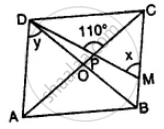# The Given Figure Shows a Rhombus Abcd in Which Angle Bcd = 80°. Find Angles X and Y. - Mathematics

Sum

The given figure shows a rhombus ABCD in which angle BCD = 80°. Find angles x and y.#### Solution

In rhombus ABCD, diagonals AC and BD bisect each other at 90°

∠BCD = 80°

Diagonals bisect the opposite angles also ∠BCD =

∠BAD = 80° and ∠ABC = ∠ADC = 180° – 80° = 100°

Diagonals bisect opposite angles

∠OCB or ∠PCB =80^circ/2 = 40°

In ∆PCM,

Ext. CPD = ∠OCB + ∠PMC

110° = 40° + x

⇒ x = 110° – 40° = 70°

and ∠ADO = 1/2 ∠ADC = 1/2 xx 100^circ = 50^circ

Hence x = 70° and y = 50°

Is there an error in this question or solution?

#### APPEARS IN

Selina Concise Mathematics Class 8 ICSE
Chapter 17 Special Types of Quadrilaterals
Exercise 17 | Q 19 | Page 199